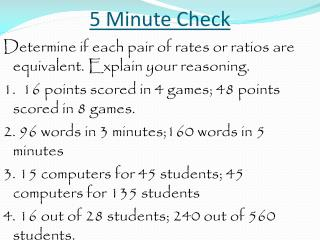Download Presentation5 Minute Check

# 5 Minute Check - PowerPoint PPT Presentation

5 Minute Check. Determine if each pair of rates or ratios are equivalent. Explain your reasoning. 1. 16 points scored in 4 games; 48 points scored in 8 games. 2. 96 words in 3 minutes;160 words in 5 minutes 3. 15 computers for 45 students; 45 computers for 135 studentsI am the owner, or an agent authorized to act on behalf of the owner, of the copyrighted work described.
Download Presentation## 5 Minute Check

An Image/Link below is provided (as is) to download presentation

Download Policy: Content on the Website is provided to you AS IS for your information and personal use and may not be sold / licensed / shared on other websites without getting consent from its author.While downloading, if for some reason you are not able to download a presentation, the publisher may have deleted the file from their server.

- - - - - - - - - - - - - - - - - - - - - - - - - - E N D - - - - - - - - - - - - - - - - - - - - - - - - - -
Presentation Transcript
1. 5 Minute Check Determine if each pair of rates or ratios are equivalent. Explain your reasoning. 1. 16 points scored in 4 games; 48 points scored in 8 games. 2. 96 words in 3 minutes;160 words in 5 minutes 3. 15 computers for 45 students; 45 computers for 135 students 4. 16 out of 28 students; 240 out of 560 students.

2. 5 Minute Check Determine if each pair of rates or ratios are equivalent. Explain your reasoning. 1. 16 points scored in 4 games; 48 points scored in 8 games. 16 points ? 48 points 4 games = 8 games 4 points6 points 1 game ≠ 1 game

3. 5 Minute Check Determine if each pair of rates or ratios are equivalent. Explain your reasoning. 2. 96 words in 3 minutes;160 words in 5 minutes.

4. 5 Minute Check Determine if each pair of rates or ratios are equivalent. Explain your reasoning. 2. 96 words in 3 minutes;160 words in 5 minutes. 96 words ? 160 words 3 minutes = 5 minutes 32 words32 words 1 minute =1 minute

5. 5 Minute Check Determine if each pair of rates or ratios are equivalent. Explain your reasoning. 3. 15 computers for 45 students; 45 computers for 135 students.

6. 5 Minute Check Determine if each pair of rates or ratios are equivalent. Explain your reasoning. 3. 15 computers for 45 students; 45 computers for 135 students. 15 computers ? 45 computers 45 students = 135 students 1 computer1 computer 3 students = 3 students

7. 5 Minute Check Determine if each pair of rates or ratios are equivalent. Explain your reasoning. 4. 16 out of 28 students; 240 out of 560 students.

8. 5 Minute Check Determine if each pair of rates or ratios are equivalent. Explain your reasoning. 4. 16 out of 28 students; 240 out of 560 students. 16 ? 240 28 = 560 4 3 7 ≠ 7

9. Inquiry Lab Turn to page 67 in your text

10. Inquiry Lab

11. Inquiry Lab

12. Inquiry Lab

13. Inquiry Lab

14. Inquiry Lab

15. Inquiry Lab

16. Inquiry Lab

17. Inquiry Lab

18. Inquiry Lab

19. Inquiry Lab

20. Inquiry Lab

21. Inquiry Lab

22. Inquiry Lab

23. Inquiry Lab

24. Inquiry Lab

25. Inquiry Lab

26. Inquiry Lab

27. Inquiry Lab

28. Inquiry Lab

29. Inquiry Lab

30. Inquiry Lab

31. Inquiry Lab Work with a partner to complete page 69 and 70. You have 15 minutes.

32. Tuesday, Sept 2 Lesson 6.1.7 Rate and Ratio Problems

33. Rate and Ratio Problems Objective: To solve rate and ratio problems.

34. Rate and Ratio Problems You can use bar diagrams, rate or ratio tables, or equivalent ratios to solve rate and ratio problems.

35. Rate and Ratio Problems

36. Rate and Ratio Problems Method 1 Make a rate table. A rate table is the same as a ratio table.

37. Rate and Ratio Problems

38. Rate and Ratio Problems

39. Rate and Ratio Problems x 50 x 50

40. Rate and Ratio Problems x 50 x 50

41. Rate and Ratio Problems Method 2 Set up equivalent rates or ratios. Rule – When setting up equivalent rates or ratios, always include units.

42. Rate and Ratio Problems Rule – When setting up equivalent rates or ratios, always include units. In the above problem, what are our units?

43. Rate and Ratio Problems ? gel ? gel ? total = ? total

44. Rate and Ratio Problems 2 gel ? gel 3 total = 150 total

45. Rate and Ratio Problems To Solve Rate and Ratio Problems by Cross Multiplying. Step 1 – Multiply across the rate or ratio. 2 gel ? gel 3 total = 150 total2 x 150 = 300

46. Rate and Ratio Problems To Solve Rate and Ratio Problems by Cross Multiplying. Step 2 – Divide by the remaining number. 2 gel? gel 3 total =150 total 2 x 150 = 300 ÷ 3 = 100

47. Rate and Ratio Problems 2? 3 = 150 2 x 150 = 300 ÷ 3 = 100 100 students would prefer gel toothpaste.

48. Rate and Ratio Problems What are our units?

49. Rate and Ratio Problems What are our units? ? Lucas texts? Lucas texts ? Sister texts = ? Sister texts